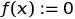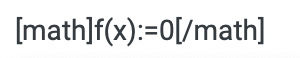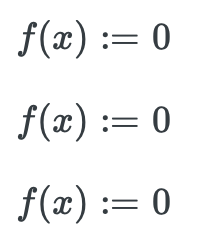# math test

## Recommended Posts

Just trying $for the first time... (Vfy)^2=(VisinΘ)^2+2ay⋅Δy [math] V_{fy}^{2} = V*sin( \phi )^{2}*2a_{y}* \Delta _{y}$

Edited by Ghideon
##### Share on other sites
• 9 months later...
• 9 months later...

1: PNG:2: Plain text: f(x):=0

3: text between math brackets: $f(x):=0$

4: Screenshot of line 3 above before pushing submit:Edited by Ghideon
##### Share on other sites

Three different modes tested:

$f(x):=0$

$$f(x):=0$$

$f(x):=0$

Code for above is

$f(x):=0$

$$f(x):=0$$

$f(x):=0$

Screendump from browserMathjax-config:

inlineMath: [ ["$math$","$\/math$"], ["\$$","\$$"], ["$latex$","$\/latex$"] ],

Edited by Ghideon
##### Share on other sites
• 9 months later...

Testing inlined math using hint from @joigus*, square brackets vs parentheses.

Sentence with three definitions $f(x^{2})=\frac{y}{2}$ $$f(x^{2})=\frac{y}{2}$$ $f(x^{2})=\frac{y}{2}$ end of sentence.

Sentence with no math.

New sentence $f(x^{2})=\frac{y}{2}$ $f(x^{2})=\frac{y}{2}$ $f(x^{2})=\frac{y}{2}$ end of sentence.

<end of test>

Below is the code for the above

Sentence with three definitions $f(x^{2})=\frac{y}{2}$ $$f(x^{2})=\frac{y}{2}$$ $f(x^{2})=\frac{y}{2}$ end of sentence.

Sentence with no math.

New sentence $f(x^{2})=\frac{y}{2}$ $f(x^{2})=\frac{y}{2}$ $f(x^{2})=\frac{y}{2}$ end of sentence.

*) Thanks !

Edited by Ghideon
##### Share on other sites

$CaC{O_3}\xrightarrow[{heat}]{}CaO + C{O_2}$

Displaying the command text in AMS Latex works if you omit the first backslash or put it between < >

[CaC{O_3}\xrightarrow[{heat}]{}CaO + C{O_2}\]

<\>[CaC{O_3}\xrightarrow[{heat}]{}CaO + C{O_2}\]

Edited by studiot
##### Share on other sites
55 minutes ago, studiot said:

Displaying the command text in AMS Latex works if you omit the first backslash or put it between < >

That's nice. Only thing is that you're forced to leave out the braces, which maybe you want to make explicit. But you gave me an idea. Let's see if it works:

$\textrm{ \[ \frac{a}{b}$ } \]

$\text{ \[ \frac{a}{b}$ } \]

Look at that. It does...

You only have to escape the math mode inside the LateX with the \textrm{} or \text{} commands.

##### Share on other sites

$\frac{a}{b}$

23 hours ago, joigus said:

$\frac{a}{b}$

$\frac{a}{b}$

Yes it seems to be copy and pastable.

I say that as I try to provide something that does not generate an error code here but can be copy/pasted rather than typed in.

##### Share on other sites
On 1/10/2021 at 6:24 PM, Ghideon said:

Below is the code for the above

You don't need brackets {} if superscript or subscript has just one character. e.g. x^2 instead of x^{2}. Subscript you make using _ character.

$x^2$

$x_a$

Edited by Sensei
##### Share on other sites
14 hours ago, studiot said:

Yes it seems to be copy and pastable.

Yes it is copy and pastable, but sadly I now note that it did not produce my intended effect.

Yes I copy.pasted from your post and it reproduced the listing of your code as you have shown it,

But MathJax the Mangler  later intervened and now my copy comes out showing the action (ie the fraction) not the code.

##### Share on other sites
16 minutes ago, studiot said:

Yes it is copy and pastable, but sadly I now note that it did not produce my intended effect.

Yes I copy.pasted from your post and it reproduced the listing of your code as you have shown it,

But MathJax the Mangler  later intervened and now my copy comes out showing the action (ie the fraction) not the code.

It interacts badly with the round brackets also when you want to show the inline maths tags. It may interact badly with other tags too. I think the safest thing when you want to show code is the code button on the toolbar.

##### Share on other sites
• 2 weeks later...

Just testing: $$a^2+b^2=c^2$$

## Create an account

Register a new account